# (2) (a) For any O E [ 0 21] let -sino Cose x For Cosce sino...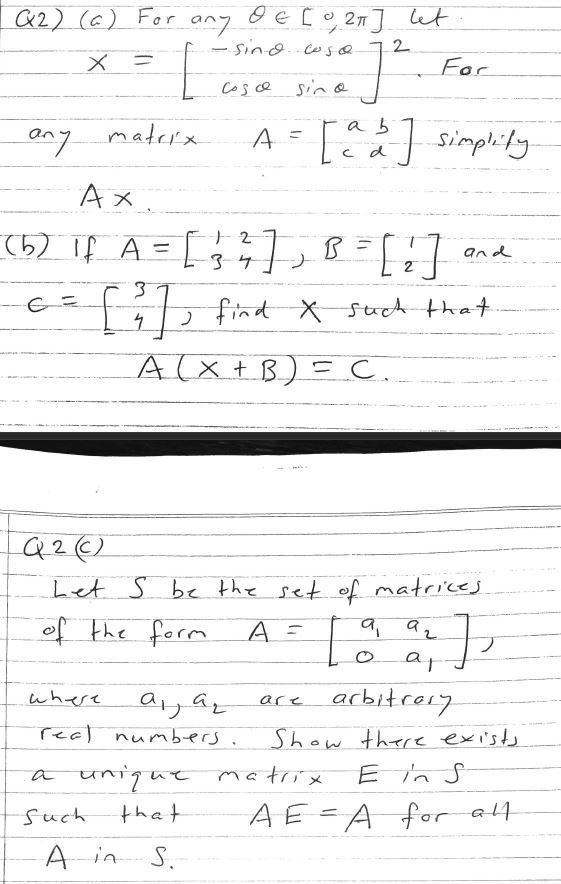(2) (a) For any O E [ 0 21] let -sino Cose x For Cosce sino 1² [ a b ] simplity any matrix A АХ 052 If A = and ... B = C], find X-sored that A(x+B) = C. Q 2 (C) Let S be the set of matrices of the form As a a2 ag where arbitrary real numbers. Show there exists a unique matrix E in s such that A EA for all o in وگرنه are S.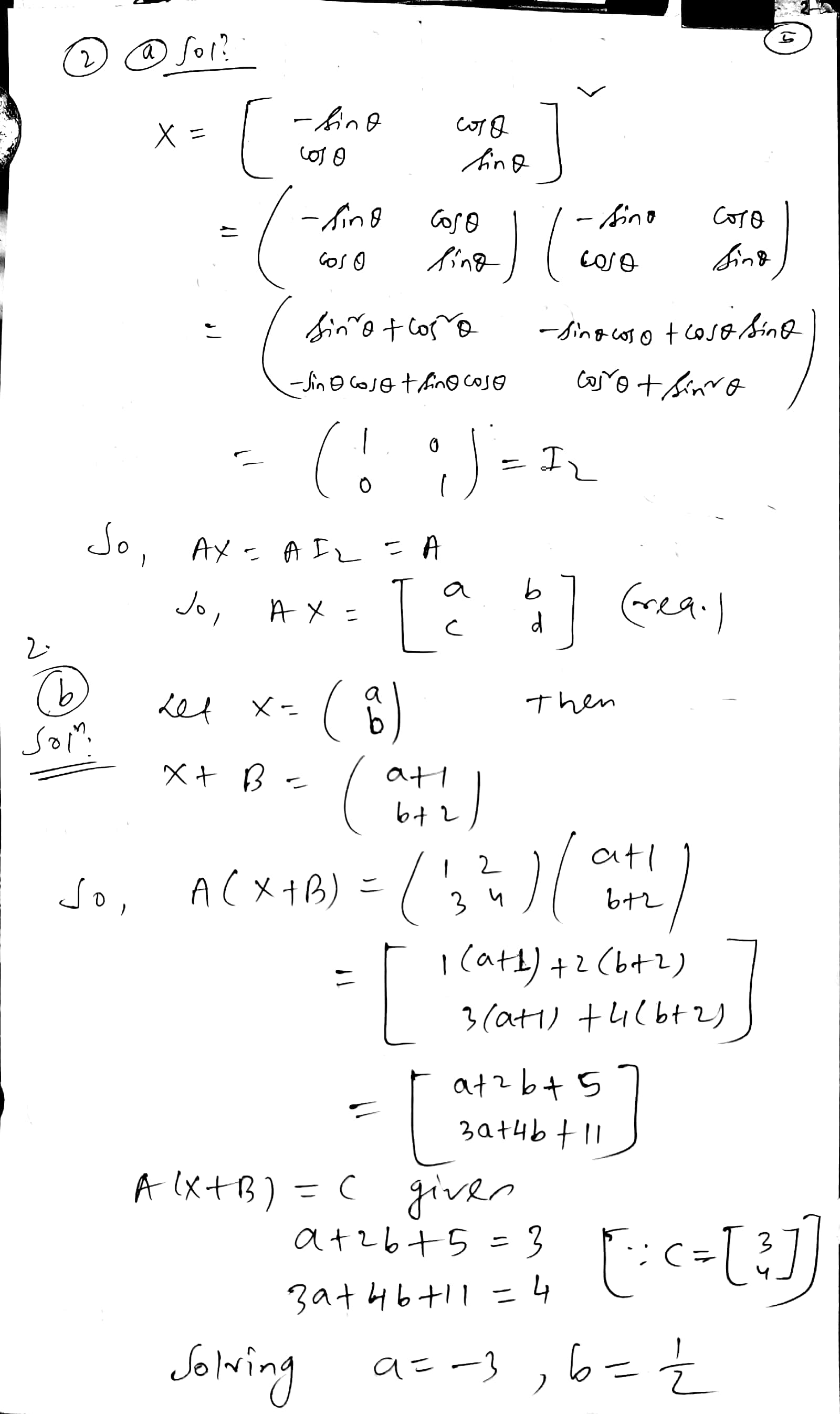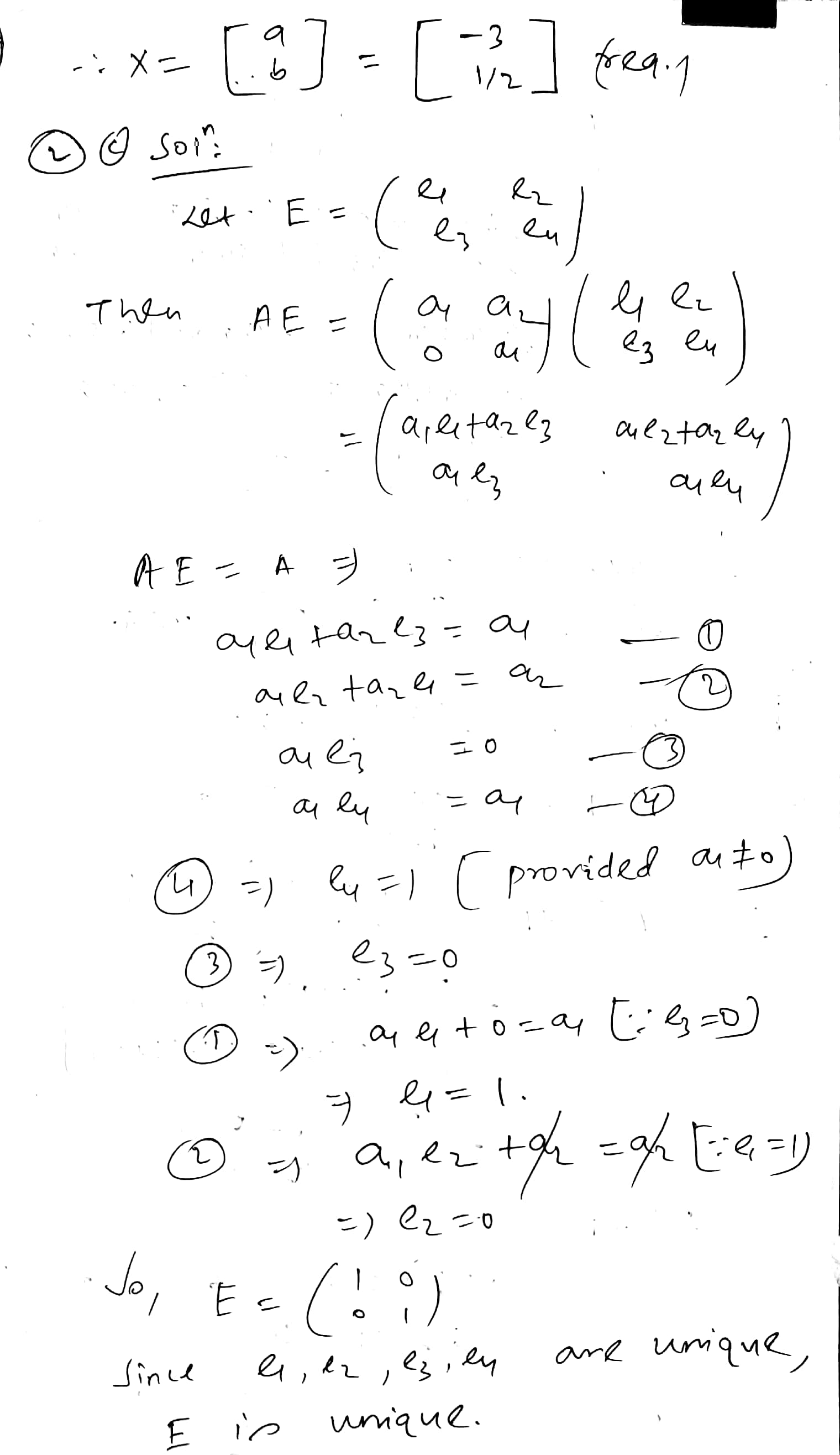##### Add Answer of: (2) (a) For any O E [ 0 21] let -sino Cose x For Cosce sino...
Similar Homework Help Questions
• ### Q 2 (c) Let S be the set of matrices of the form A = a,...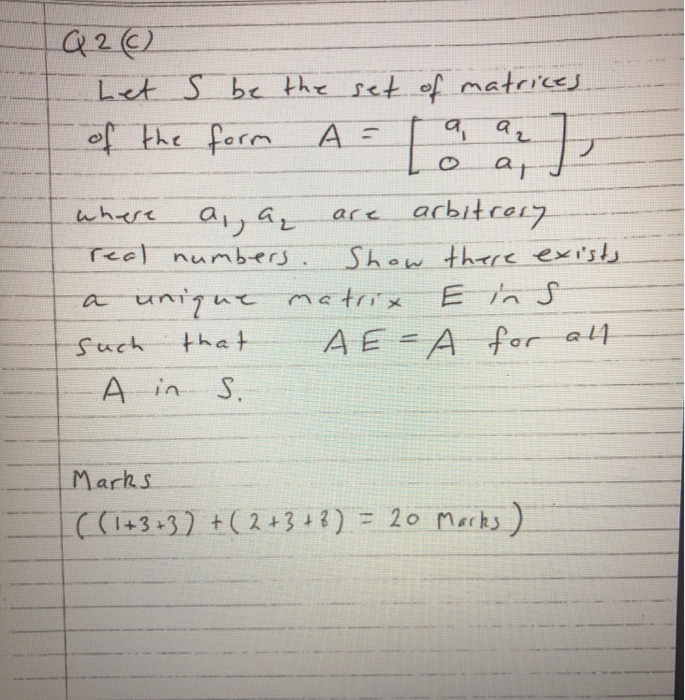Q 2 (c) Let S be the set of matrices of the form A = a, a T ag arbitrosy where are real numbers. Show there exists a unique matrix E in s such that АЕА o in S. for all Marks ((1+3+37 +(2+3 + 8) = 20 Marks) MATH 2118 Online Class Exercise I Qla) Sketch the surface s defined by the equation z = =9-6tty! (6) Determine the equation of the tongent plane to the surface s given...

• ### O Q 2 (C) Let S be the set of matrices of the form A= a...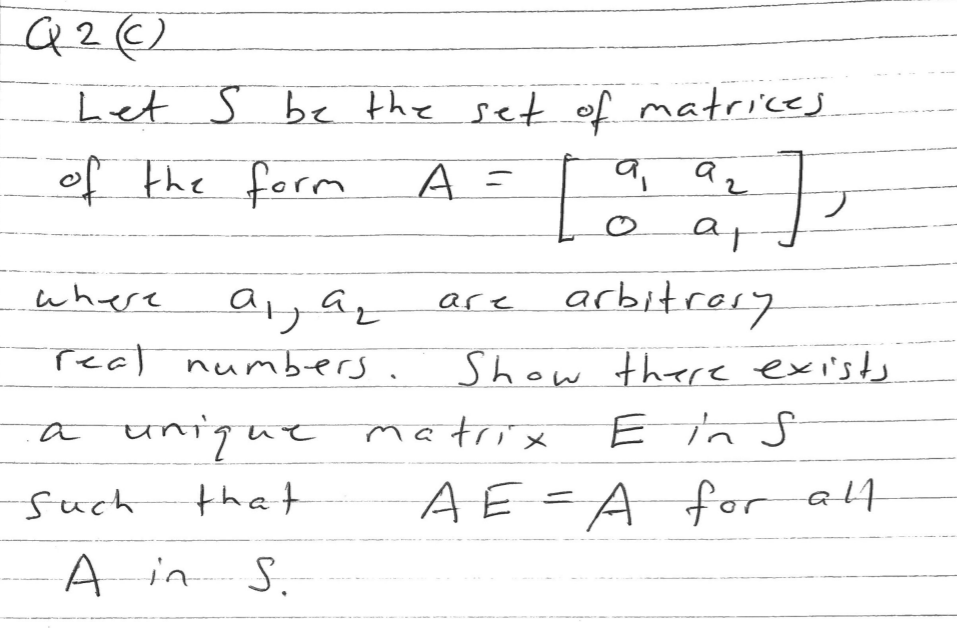O Q 2 (C) Let S be the set of matrices of the form A= a az ag where a ja, are arbitrary real numbers. Show there exists a unique matrix such that A EA for all A in S.

• ### A) 2 For cos 23 -sino cos For sino any matrix A = any € [0,...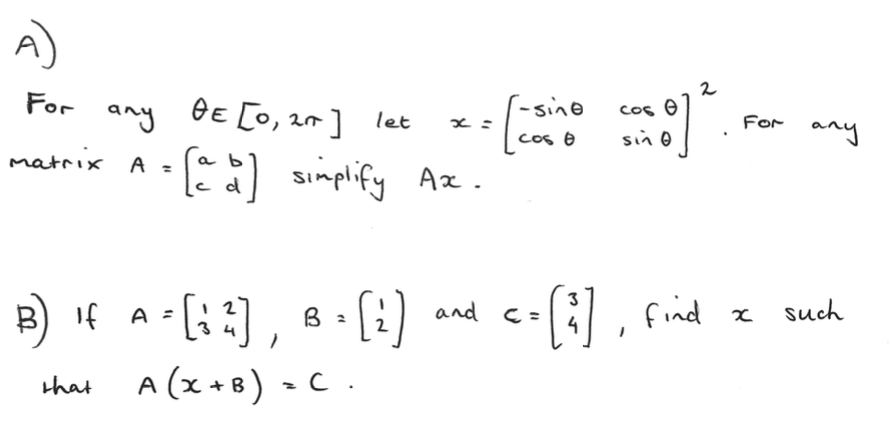A) 2 For cos 23 -sino cos For sino any matrix A = any € [0, 21] let [a b] simplify simplify Ax. B) If A = B) and c Coff 8 , find 2 A- , B = A (x +B) =(. such that

• ### Problem 5. Let n N. The goal of this problem is to show that if two real n x n matrices are simil...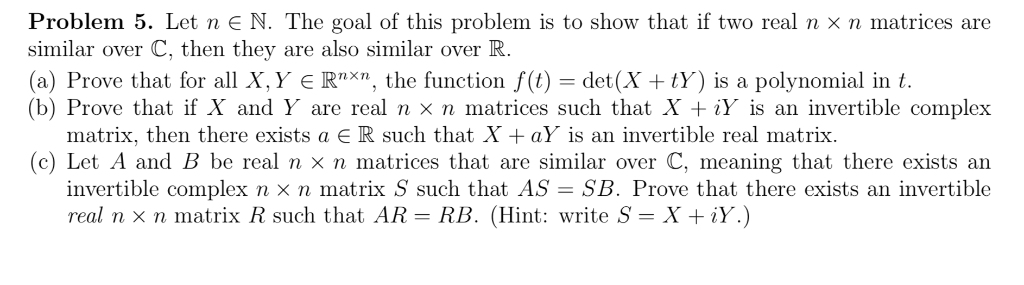Problem 5. Let n N. The goal of this problem is to show that if two real n x n matrices are similar over C, then they are also similar over IK (a) Prove that for all X, y є Rnxn, the function f(t) det (X + ty) is a polynomial in t. (b) Prove that if X and Y are real n × n matrices such that X + ừ is an invertible complex matrix, then there exists a...

• ### Question 8. a) Let I be the n x n identity matrix and let O be...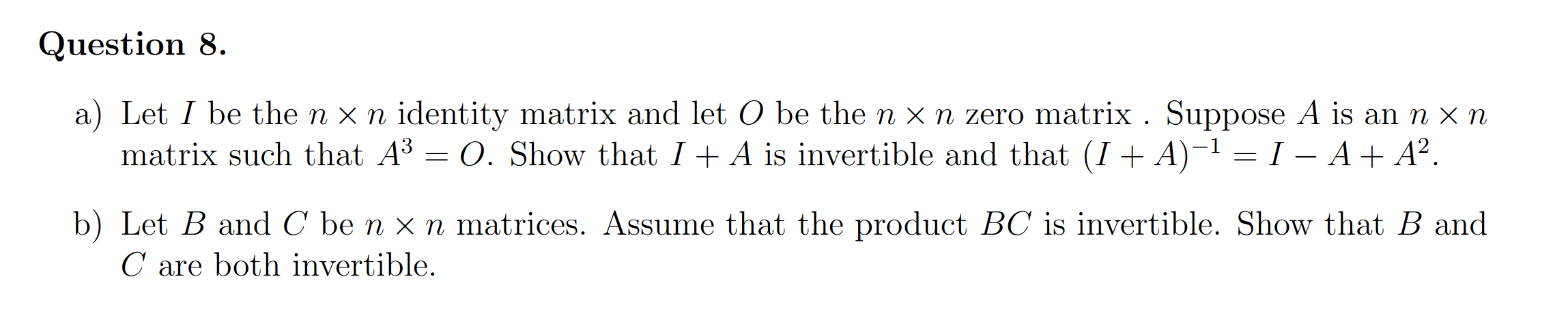Question 8. a) Let I be the n x n identity matrix and let O be the n x n zero matrix . Suppose A is an n xn matrix such that A3 = 0. Show that I + A is invertible and that (I + A)-1 = I – A+ A2. b) Let B and C be n x n matrices. Assume that the product BC is invertible. Show that B and C are both invertible.

• ### #21. Let G be the set of all real 2 x 2 matrices where ad +...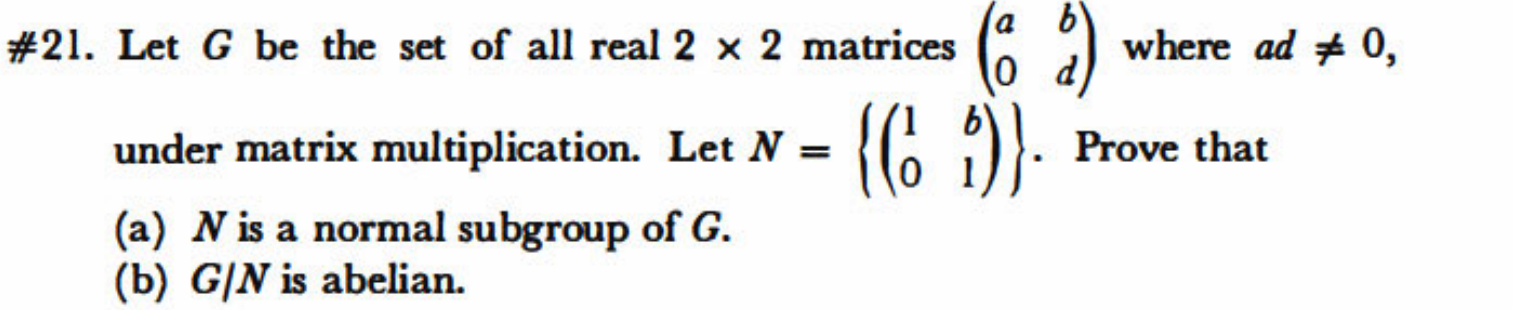#21. Let G be the set of all real 2 x 2 matrices where ad + 0, Prove that under matrix multiplication. Let N = (a) N is a normal subgroup of G. (b) G/N is abelian.

• ### 3. Let a >0, and for any A E Rnxn, define Aa aA (a) Prove that for any induced matrix norm, K(Ao)...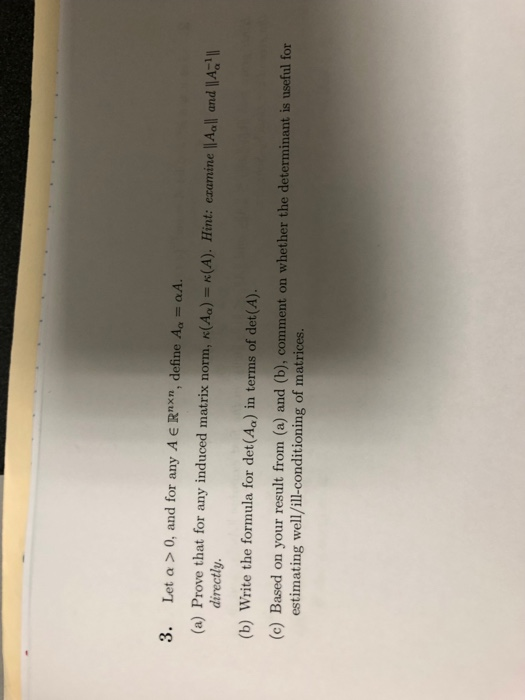3. Let a >0, and for any A E Rnxn, define Aa aA (a) Prove that for any induced matrix norm, K(Ao) (b) Write the formula for det(Aa) in terms of det(A). estimating well/ill-conditioning of matrices. n(A) . Hint: examine IAall and IAal directly. (c) Based on your result from (a) and (b), comment on whether the determinant is useful for 3. Let a >0, and for any A E Rnxn, define Aa aA (a) Prove that for any induced...

• ### How to do Part 3? -- Find e^(At), the exponential of matrix A, where t ∈ ℝ is any real number.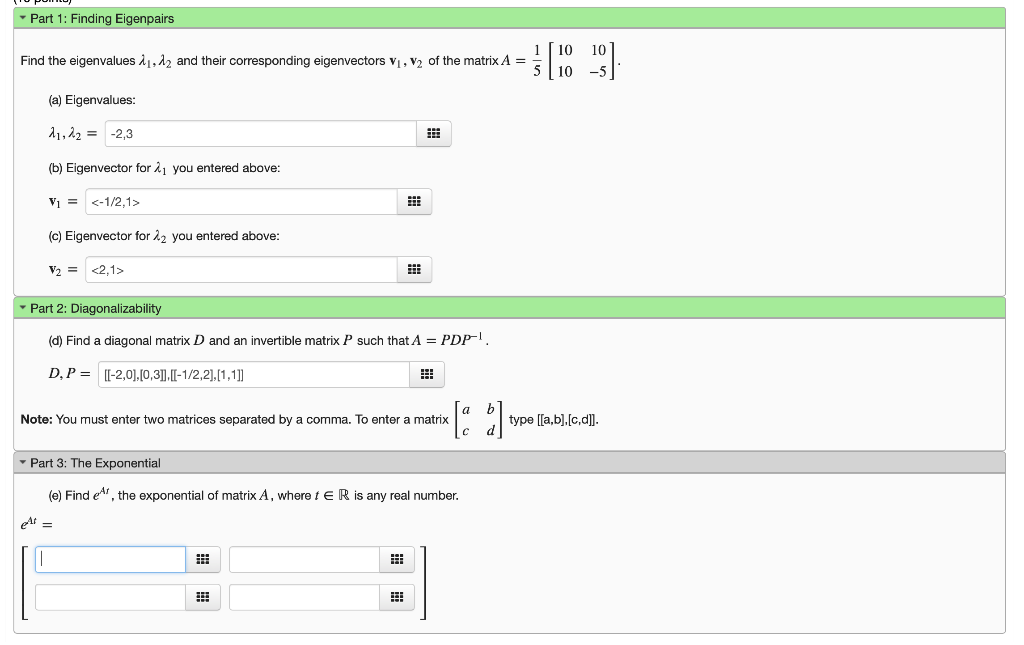How to do Part 3? -- Find e^(At), the exponential of matrix A, where t ∈ ℝ is any real number. Part 1: Finding Eigenpairs [10 10 5 10 -5 Find the eigenvalues λ,A2 and their corresponding eigenvectors vi , v2 of the matrix A- (a) Eigenvalues: 1,222.3 (b) Eigenvector for 21 you entered above: Vi = <-1/2,1> (c) Eigenvector for 22 you entered above: Part 2: Diagonalizability (d) Find a diagonal matrix D and an invertible matrix P D,...

• ### 5. Let B be the following matrix in reduced row-echelon form: 1 B= 1 -1 0-1...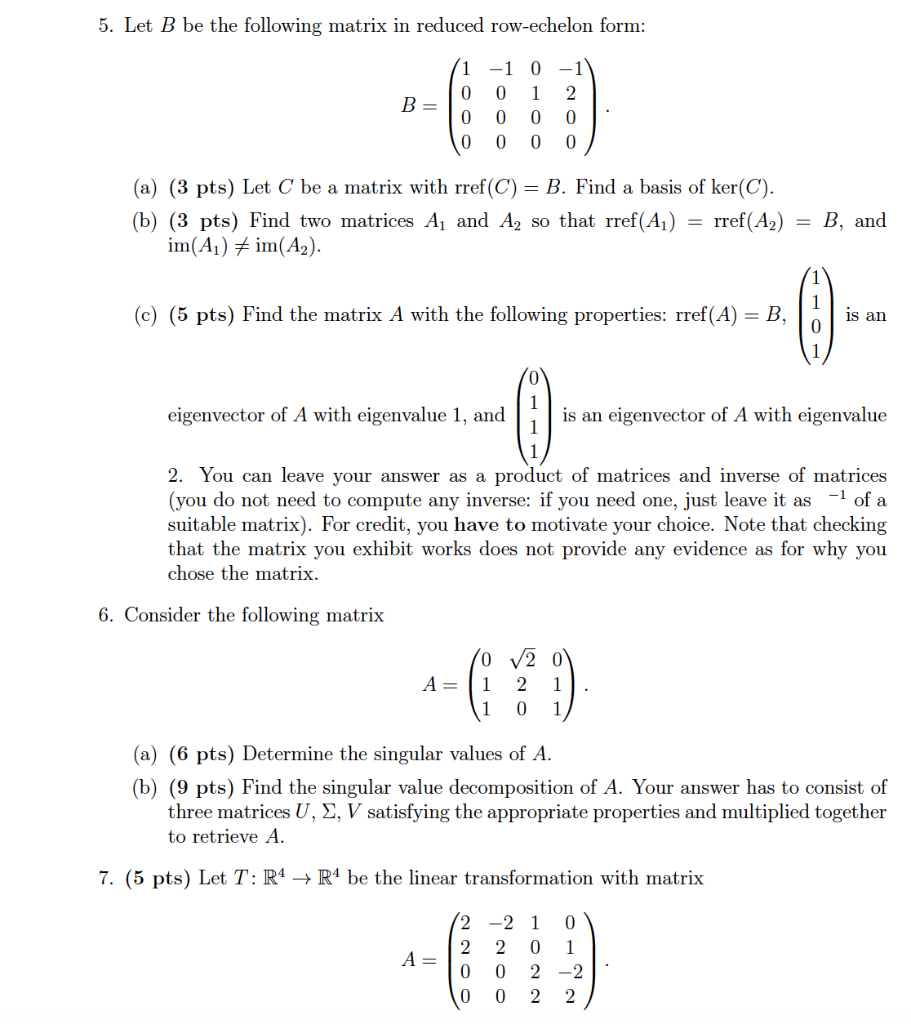5. Let B be the following matrix in reduced row-echelon form: 1 B= 1 -1 0-1 0 0 2 0 0 0 0 0 0 0 0 (a) (3 pts) Let C be a matrix with rref(C) = B. Find a basis of ker(C). (b) (3 pts) Find two matrices A1 and A2 so that rref(A1) = rref(A2) im(A) # im(A2). B, and 1 (c) (5 pts) Find the matrix A with the following properties: rref(A) = B, is an...

• ### Problem 3. Let V and W be vector spaces of dimensions n and m, respectively, and let T : V -> V be a linear transfor...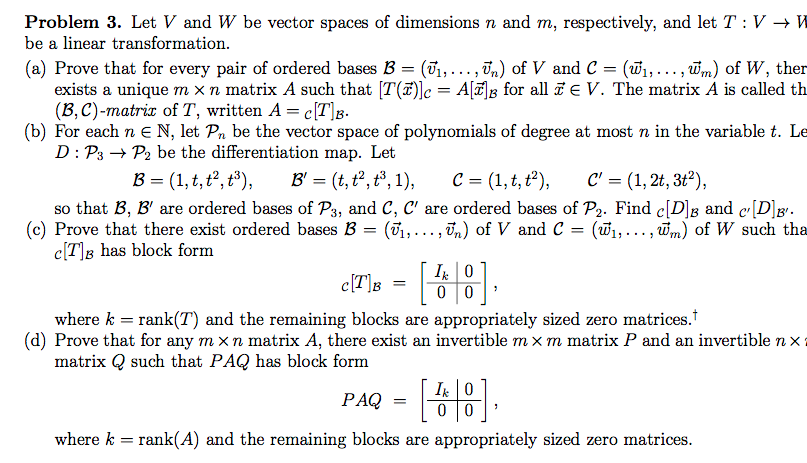Problem 3. Let V and W be vector spaces of dimensions n and m, respectively, and let T : V -> V be a linear transformation (a) Prove that for every pair of ordered bases B = (Ti,...,T,) of V and C = (Wi, ..., Wm) of W, then exists a unique (B, C)-matrix of T, written A = c[T]g. (b) For each n e N, let Pn be the vector space of polynomials of degree at mostn in the...

Free Homework App